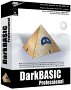# Physics - Derivation of Inertia Tensor

To derive the expression for the inertia tensor lets calculate the angular velocity due to a torque for a small cube of matter and then integrate this over the whole mass of the object.

However, there is a problem, how do we apply a torque to one point only? I thought of having two small cubes on opposite sides of the centre of mass but this would only work if the object were symmetrical. So instead I will pin the centre of mass to the origin, because this is the centre of mass when we integrate the forces on the origin should cancel out?So we take a small volume (Δx,Δy,Δz) at the point (rx,ry,rz) which has the mass Δm

How do we apply a force couple to generate the required torque?

The inertia tensor represents the relationship between the torque and the angular acceleration as follows:

T = [I]α

Or alternativly, the relationship between the angular momentum and the angular velocity, we will use this:

L = angular momentum = [I]ω

we can represent the angular momentum of the small particle as its linear velocity multiplied (cross product) by its distance from the centre of rotation. So integrating over all the particles gives:

L = ∫ r × v dm

but the linear velocity v = ω × r, substituting this gives:

L = ∫ r × (ω × r) dm

We can use this result using geometric algebra or we and convert to the more usual matrix form by replacing these vectors with a screw symmetric matrix as follows:

## Matrix Representation

r × (ω × r)

= -r × (r ×ω)

= -[~r][~r]ω

I = - ∫
 0 - rz ry rz 0 - rx -ry rx 0
 0 - rz ry rz 0 - rx -ry rx 0
dm
I = - ∫
 -rz²-ry² rx*ry rx*rz ry*rx -rz²-rx² ry*rz rz*rx rz*ry -rx²-ry²
dm
I =
 ∫(rz²+ry²)dm -∫rx*rydm -∫rx*rzdm -∫ry*rxdm ∫(rz²+rx²)dm -∫ry*rzdm -∫rz*rxdm -∫rz*rydm ∫(rx²+ry²)dm

Where I can, I have put links to Amazon for books that are relevant to the subject, click on the appropriate country flag to get more details of the book or to buy it from them.Engineering Mechanics Vol 2: Dynamics - Gives theory for rigid dynamics, aims to allow prediction of effects of force and motion. Includes rotating frame of reference. Lots of colour diagrams, I guess its college / University level.

Commercial Software Shop

Where I can, I have put links to Amazon for commercial software, not directly related to the software project, but related to the subject being discussed, click on the appropriate country flag to get more details of the software or to buy it from them.Dark Basic Professional Edition - It is better to get this professional editionThis is a version of basic designed for building games, for example to rotate a cube you might do the following: make object cube 1,100 for x=1 to 360 rotate object 1,x,x,0 next x

This site may have errors. Don't use for critical systems.